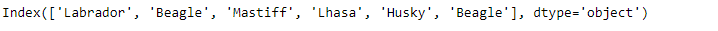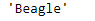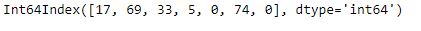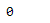# Python | Pandas Index.min()

Python is a great language for doing data analysis, primarily because of the fantastic ecosystem of data-centric python packages. Pandas is one of those packages and makes importing and analyzing data much easier.

Pandas` Index.min()` function returns the minimum value of the Index. The function works with both numerical as well as the string type objects. In case of string type object it returns the string which has smallest value in lexicographical order.

Syntax: Index.min()

Parameters : Doesn’t take any parameter.

Returns : scalar: Minimum value.

Example #1: Use `Index.min()` function to find the minimum element in the given Index.

 `# importing pandas as pd ` `import` `pandas as pd ` ` `  `# Creating the Index ` `idx ``=` `pd.Index([``'Labrador'``, ``'Beagle'``, ``'Mastiff'``, ``'Lhasa'``, ``'Husky'``, ``'Beagle'``]) ` ` `  `# Print the Index ` `idx `

Output :Now we find the min value in the given Index.

 `# return min value. ` `idx.``min``() `

Output :As we can see in the output, the function has returned ‘Beagle’ which has the smallest lexicographical order among the values present in the Index.

Example #2: Use `Index.min()` function to find the minimum value in the Index.

 `# importing pandas as pd ` `import` `pandas as pd ` ` `  `# Creating the Index ` `idx ``=` `pd.Index([``17``, ``69``, ``33``, ``5``, ``0``, ``74``, ``0``]) ` ` `  `# Print the Datetime Index ` `idx `

Output :Now we will find the minimum value among the labels of the Index.

 `# the function will return the minimum ` `#  value present in the Index ` `idx.``min``() `

Output :As we can see in the output, the function has returned 0 which is the smallest value among the labels of the Index.

My Personal Notes arrow_drop_upCheck out this Author's contributed articles.

If you like GeeksforGeeks and would like to contribute, you can also write an article using contribute.geeksforgeeks.org or mail your article to contribute@geeksforgeeks.org. See your article appearing on the GeeksforGeeks main page and help other Geeks.

Please Improve this article if you find anything incorrect by clicking on the "Improve Article" button below.

Article Tags :

Be the First to upvote.

Please write to us at contribute@geeksforgeeks.org to report any issue with the above content.